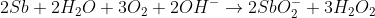# AP Chemistry : Balancing Oxidation-Reduction Reactions

## Example Questions

### Example Question #1 : Balancing Oxidation Reduction Reactions

The following ReDox reaction takes place in acidic solution:

Fe2+ + Cr2O72– → Fe3+ + Cr3+

What is the sum of coefficients in this redox reaction?

37

33

34

36

35

36

Explanation:

When you balance the redox reaction in acidic conditons, there are 6Fe2+, 1 Cr2O72–, 14 H+, 6 Fe3+, 2 Cr3+, and 7 H2O. Don't forget to add the 1 in front of the Cr2O72–

### Example Question #2 : Balancing Oxidation Reduction Reactions

For the following unbalanced redox reaction, how many electrons are transferred and which chemical species is being oxidized?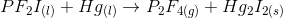One electron is transferred; P is oxidized

Two electrons are transferred; P is oxidized

One electron is transferred; Hg is oxidized

Two electrons are transferred; Hg is oxidized

Two electrons are transferred; Hg is oxidized

Explanation:

To begin, we will need to separate the given reaction into the two half-reactions by identifying changes in oxidation number. In this case, mercury (Hg) and phosphorus (P) show a change in oxidation number. Mercury begins with an oxidation number of zero, and ends with an oxidation number of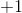. Phosphorus begins with an oxidation number of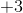and ends with an oxidation number of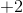. Note that the oxidation numbers for fluorine and iodine reamain constant atfor each.

Now we can begin to look at the half-reactions.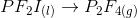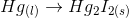Balance the atoms.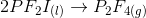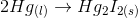Now balance the electrons. We know that each mercury atom loses one electron and each fluorine atom gains one electron.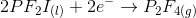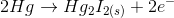We can see that two electrons are tranferred. To identify the element being oxidized, we must find the element that is losing electrons. In this case, mercury is being oxidized.

### Example Question #2 : Balancing Oxidation Reduction Reactions

How many electrons are involved in the following reaction?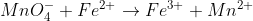1 e-

2 e-

4 e-

5 e-

3 e-

5 e-

Explanation: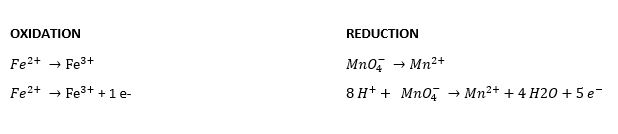The common factor between 1 e- and 5 e- is 5.  Therefore the number of electrons involved is 5 e-.

### Example Question #3 : Balancing Oxidation Reduction Reactions

How many electrons are involved in the following reaction?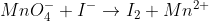4 e-

1 e-

2 e-

10 e-

5 e-

10 e-

Explanation: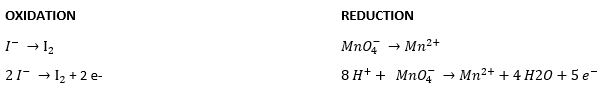The common factor between 2 e- and 5 e- is 10.  Therefore the number of electrons involved is 10 e-.

### Example Question #4 : Balancing Oxidation Reduction Reactions

What is the balanced coefficient on OH- for the following reaction: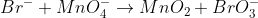(under basic conditions)

1

5

2

3

4

2

Explanation: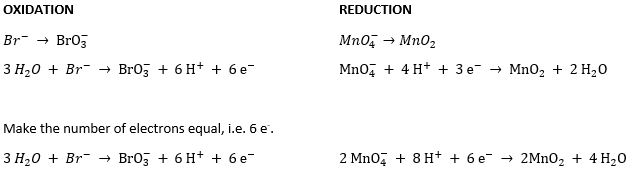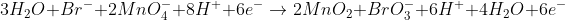Simplify: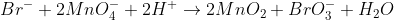Add Hydroxides to each side to counter H+.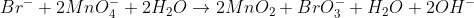Simplify: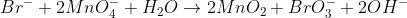### Example Question #5 : Balancing Oxidation Reduction Reactions

What is the sum of all the balanced coefficients in the following reaction: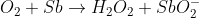(basic conditions)

10

12

8

16

14

14

Explanation: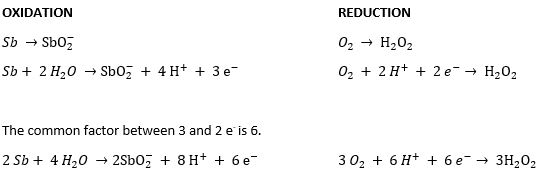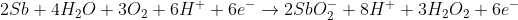Simplify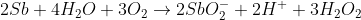Add 2 OH- to each side to cancel out the H+.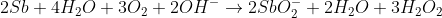Simplify: